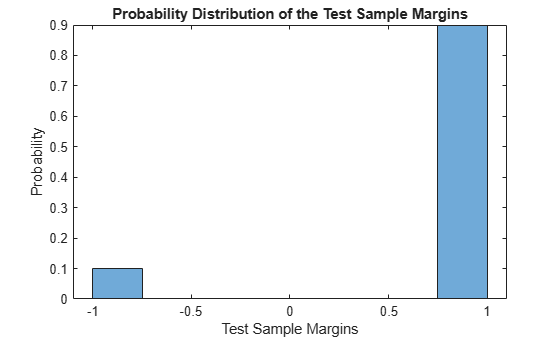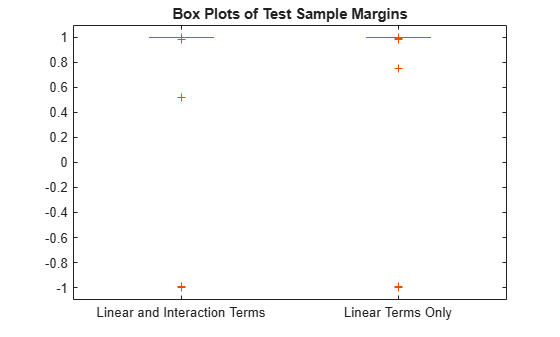margin

Classification margins for generalized additive model (GAM)

Description

m = margin(Mdl,Tbl,ResponseVarName) returns the Classification Margin (m) for the generalized additive model Mdl using the predictor data in Tbl and the true class labels in Tbl.ResponseVarName.

m is returned as an n-by-1 numeric column vector, where n is the number of observations in the predictor data.

m = margin(Mdl,Tbl,Y) uses the predictor data in table Tbl and the true class labels in Y.

example

m = margin(Mdl,X,Y) uses the predictor data in matrix X and the true class labels in Y.

example

m = margin(___,'IncludeInteractions',includeInteractions) specifies whether to include interaction terms in computations. You can specify includeInteractions in addition to any of the input argument combinations in the previous syntaxes.

Examples

collapse all

Estimate the test sample classification margins and edge of a generalized additive model. The test sample margins are the observed true class scores minus the false class scores, and the test sample edge is the mean of the margins.

Load the fisheriris data set. Create X as a numeric matrix that contains two sepal and two petal measurements for versicolor and virginica irises. Create Y as a cell array of character vectors that contains the corresponding iris species.

inds = strcmp(species,'versicolor') | strcmp(species,'virginica');
X = meas(inds,:);
Y = species(inds,:);

Randomly partition observations into a training set and a test set with stratification, using the class information in Y. Specify a 30% holdout sample for testing.

rng('default') % For reproducibility
cv = cvpartition(Y,'HoldOut',0.30);

Extract the training and test indices.

trainInds = training(cv);
testInds = test(cv);

Specify the training and test data sets.

XTrain = X(trainInds,:);
YTrain = Y(trainInds);
XTest = X(testInds,:);
YTest = Y(testInds);

Train a GAM using the predictors XTrain and class labels YTrain. A recommended practice is to specify the class names.

Mdl = fitcgam(XTrain,YTrain,'ClassNames',{'versicolor','virginica'});

Mdl is a ClassificationGAM model object.

Estimate the test sample classification margins and edge.

m = margin(Mdl,XTest,YTest);
e = edge(Mdl,XTest,YTest)
e = 0.8000

Display the histogram of the test sample classification margins.

histogram(m,length(unique(m)),'Normalization','probability')
xlabel('Test Sample Margins')
ylabel('Probability')
title('Probability Distribution of the Test Sample Margins')Compare a GAM with linear terms to a GAM with both linear and interaction terms by examining the test sample margins and edge. Based solely on this comparison, the classifier with the highest margins and edge is the best model.

Load the ionosphere data set. This data set has 34 predictors and 351 binary responses for radar returns, either bad ('b') or good ('g').

Randomly partition observations into a training set and a test set with stratification, using the class information in Y. Specify a 30% holdout sample for testing.

rng('default') % For reproducibility
cv = cvpartition(Y,'Holdout',0.30);

Extract the training and test indices.

trainInds = training(cv);
testInds = test(cv);

Specify the training and test data sets.

XTrain = X(trainInds,:);
YTrain = Y(trainInds);
XTest = X(testInds,:);
YTest = Y(testInds);

Train a GAM that contains both linear and interaction terms for predictors. Specify to include all available interaction terms whose p-values are not greater than 0.05.

Mdl = fitcgam(XTrain,YTrain,'Interactions','all','MaxPValue',0.05)
Mdl =
ClassificationGAM
ResponseName: 'Y'
CategoricalPredictors: []
ClassNames: {'b'  'g'}
ScoreTransform: 'logit'
Intercept: 3.0398
Interactions: [561x2 double]
NumObservations: 246

Properties, Methods

Mdl is a ClassificationGAM model object. Mdl includes all available interaction terms.

Estimate the test sample margins and edge for Mdl.

M = margin(Mdl,XTest,YTest);
E = edge(Mdl,XTest,YTest)
E = 0.7848

Estimate the test sample margins and edge for Mdl without including interaction terms.

M_nointeractions = margin(Mdl,XTest,YTest,'IncludeInteractions',false);
E_nointeractions = edge(Mdl,XTest,YTest,'IncludeInteractions',false)
E_nointeractions = 0.7871

Display the distributions of the margins using box plots.

boxplot([M M_nointeractions],'Labels',{'Linear and Interaction Terms','Linear Terms Only'})
title('Box Plots of Test Sample Margins')The margins M and M_nointeractions have a similar distribution, but the test sample edge of the classifier with only linear terms is larger. Classifiers that yield relatively large margins are preferred.

Input Arguments

collapse all

Generalized additive model, specified as a ClassificationGAM or CompactClassificationGAM model object.

• If you trained Mdl using sample data contained in a table, then the input data for margin must also be in a table (Tbl).

• If you trained Mdl using sample data contained in a matrix, then the input data for margin must also be in a matrix (X).

Sample data, specified as a table. Each row of Tbl corresponds to one observation, and each column corresponds to one predictor variable. Multicolumn variables and cell arrays other than cell arrays of character vectors are not allowed.

Tbl must contain all the predictors used to train Mdl. Optionally, Tbl can contain a column for the response variable and a column for the observation weights.

• The response variable must have the same data type as Mdl.Y. (The software treats string arrays as cell arrays of character vectors.) If the response variable in Tbl has the same name as the response variable used to train Mdl, then you do not need to specify ResponseVarName.

• The weight values must be a numeric vector. You must specify the observation weights in Tbl by using 'Weights'.

If you trained Mdl using sample data contained in a table, then the input data for margin must also be in a table.

Data Types: table

Response variable name, specified as a character vector or string scalar containing the name of the response variable in Tbl. For example, if the response variable Y is stored in Tbl.Y, then specify it as 'Y'.

Data Types: char | string

Class labels, specified as a categorical, character, or string array, a logical or numeric vector, or a cell array of character vectors. Each row of Y represents the classification of the corresponding row of X or Tbl.

Y must have the same data type as Mdl.Y. (The software treats string arrays as cell arrays of character vectors.)

Data Types: single | double | categorical | logical | char | string | cell

Predictor data, specified as a numeric matrix. Each row of X corresponds to one observation, and each column corresponds to one predictor variable.

If you trained Mdl using sample data contained in a matrix, then the input data for margin must also be in a matrix.

Data Types: single | double

Flag to include interaction terms of the model, specified as true or false.

The default includeInteractions value is true if Mdl contains interaction terms. The value must be false if the model does not contain interaction terms.

Data Types: logical

collapse all

Classification Margin

The classification margin for binary classification is, for each observation, the difference between the classification score for the true class and the classification score for the false class.

If the margins are on the same scale (that is, the score values are based on the same score transformation), then they serve as a classification confidence measure. Among multiple classifiers, those that yield greater margins are better.